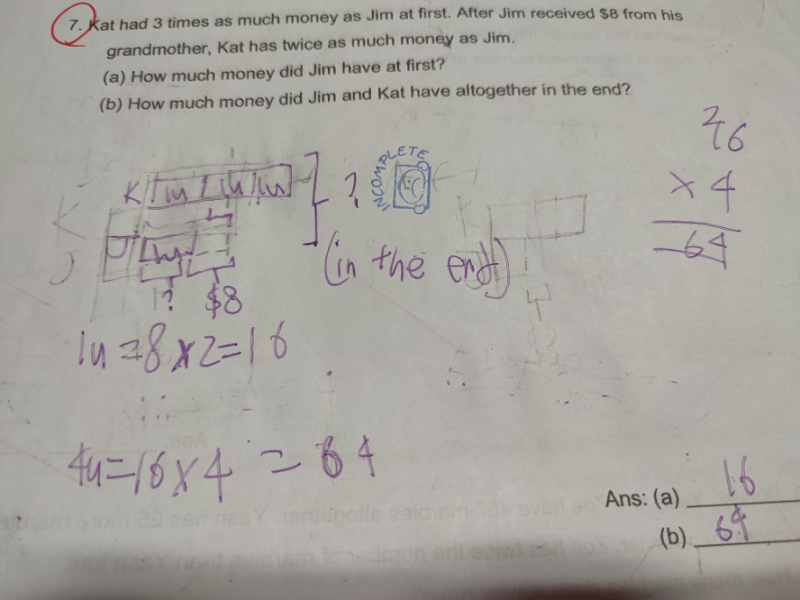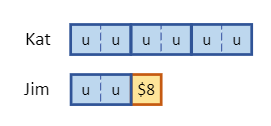# QuestionHi Parents, p4 Maths can assist to check if working and answer is correct? Thanks!

```Your drawing is correct. Part (a) is correct too.
But maybe you misread Part (b) and thought the question
is asking for how much money they both had at the start.
At the start, they both had 4 x 16 = \$64.
At the end, they both had 4 x 16 + 8 = \$72.
===```
```Here is another solution, where we use 2u for Jim's
starting amount. Then Kat will have 6u. It is clear
from the diagram below that u = \$8.```
```Part (a): Jim had 2u = 2 x 8 = \$16 at the start.
Part (b): Jim and Kat had 9u = 9 x 8 = \$72 at the end.```
` `
` `
0 Replies 3 Likes